×
INTELLIGENT WORK FORUMS
FOR ENGINEERING PROFESSIONALS

Are you an
Engineering professional?
Join Eng-Tips Forums!
• Talk With Other Members
• Be Notified Of Responses
• Keyword Search
Favorite Forums
• Automated Signatures
• Best Of All, It's Free!

*Eng-Tips's functionality depends on members receiving e-mail. By joining you are opting in to receive e-mail.

#### Posting Guidelines

Promoting, selling, recruiting, coursework and thesis posting is forbidden.

# Moving Heat Flux (laser) - From the simplest example - DFLUX subroutine4

## Moving Heat Flux (laser) - From the simplest example - DFLUX subroutine

(OP)
Hello,
I have to write the DFLUX subroutine for moving heat flux (laser, described by Gaussian equation). It should be "volumetric heat flux". I am novice in writing subroutines. It is first in my life.

Here is the equation: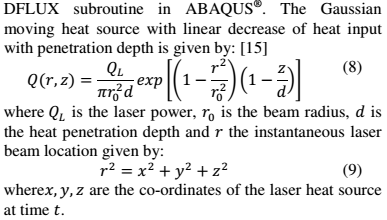And here is the figure of substrate, on which the heat flux is applied: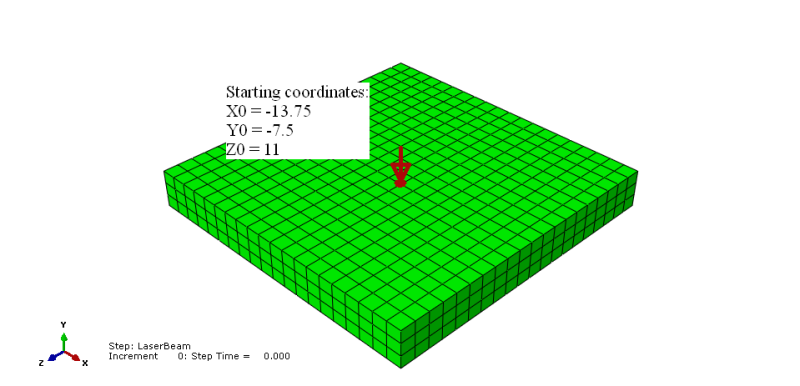Starting coordinates of the beam are (mm are used in whole model):
X0 = -13.75 [mm]
Y0 = -7.5 [mm]
Z0 = 11 [mm]

Characteristics of laser:
Scanning speeds: 4-6mm/s
Laser power: 120W
Laser spot size: 1-1.2mm at diameter
Focus distance: 5mm

DFLUX subroutine:
Because of the fact, that I do not have experience in writing subroutines, I want to start from the easiest case. First, I want to define heat flux without moving (stationary):

#### CODE --> Fortran

SUBROUTINE DFLUX(FLUX,SOL,KSTEP,KINC,TIME,NOEL,NPT,COORDS,
1 JLTYP,TEMP,PRESS,SNAME)
C
INCLUDE 'ABA_PARAM.INC'
C
DIMENSION FLUX(2), TIME(2), COORDS(3)
CHARACTER*80 SNAME

QL=120000.d0                        !LASER POWER [mW]
DEPTH=5.d0                          !PENETRATION DEPTH [mm]
Q=(QL/(R0**2*3.1415926d0*DEPTH))    !BODY FLUX [mW/mm^3] ?
V=5.d0                              !LASER BEAM VELOCITY [mm/s]

X0=-13.75d0                         !STARTING COORDINATE OF THE LASER BEAM
Y0=-7.5d0                           !STARTING COORDINATE OF THE LASER BEAM
Z0=11d0                             !STARTING COORDINATE OF THE LASER BEAM

FLUX(1)=Q

RETURN
END 

The first problem is, that the heat affect whole surface, not the coordinates X0, Y0, Z0. I suppose, that the X0, Y0 and Z0 coordinates should be included in some way into FLUX(1) line. I saw other examples of moving heat flux on the Internet, but they are too complicated for me at the moment. I want to understand the code.

Maybe someone will explain me, what should I add to the code?

### RE: Moving Heat Flux (laser) - From the simplest example - DFLUX subroutine

V, X0, Y0, Z0 are doing nothing in your subroutine. You should have some kind of IF statement that says that if the coordinates are within a range then the flux=whatever, else is zero, and where the coordinate position is dependent upon time.

### RE: Moving Heat Flux (laser) - From the simplest example - DFLUX subroutine

(OP)

The upgraded code is presented below:

#### CODE --> Fortran

SUBROUTINE DFLUX(FLUX,SOL,KSTEP,KINC,TIME,NOEL,NPT,COORDS,
1 JLTYP,TEMP,PRESS,SNAME)
C
INCLUDE 'ABA_PARAM.INC'
C
DIMENSION FLUX(2), TIME(2), COORDS(3)
CHARACTER*80 SNAME

QL=120000.d0                        !LASER POWER [mW]
DEPTH=0.5d0                         !PENETRATION DEPTH [mm]
Q=(QL/(R0**2*3.1415926d0*DEPTH))    !BODY FLUX [mW/mm^3] ?
!     V=5.d0                              !LASER BEAM VELOCITY [mm/s]

X0=-13.75d0                         !STARTING COORDINATE OF THE LASER BEAM
Y0=-7.5d0                           !STARTING COORDINATE OF THE LASER BEAM
Z0=11.d0                            !STARTING COORDINATE OF THE LASER BEAM

X=COORDS(1)
Y=COORDS(2)
Z=COORDS(3)

IF(X.EQ.X0) THEN
FLUX(1)=Q
ELSE
FLUX(1)=0
END IF

RETURN
END 

The results are: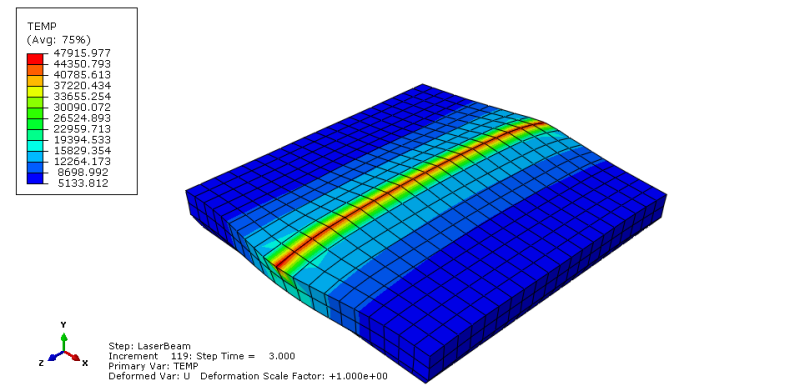It looks good, but I don't understand why the temperature values are so big.
When I try to do the same, but with Z coordinates:

#### CODE --> Fortran

SUBROUTINE DFLUX(FLUX,SOL,KSTEP,KINC,TIME,NOEL,NPT,COORDS,
1 JLTYP,TEMP,PRESS,SNAME)
C
INCLUDE 'ABA_PARAM.INC'
C
DIMENSION FLUX(2), TIME(2), COORDS(3)
CHARACTER*80 SNAME

QL=120000.d0                        !LASER POWER [mW]
DEPTH=0.5d0                         !PENETRATION DEPTH [mm]
Q=(QL/(R0**2*3.1415926d0*DEPTH))    !BODY FLUX [mW/mm^3] ?
!     V=5.d0                              !LASER BEAM VELOCITY [mm/s]

X0=-13.75d0                         !STARTING COORDINATE OF THE LASER BEAM
Y0=-7.5d0                           !STARTING COORDINATE OF THE LASER BEAM
Z0=11.d0                            !STARTING COORDINATE OF THE LASER BEAM

X=COORDS(1)
Y=COORDS(2)
Z=COORDS(3)

IF(Z.EQ.Z0) THEN
FLUX(1)=Q
ELSE
FLUX(1)=0
END IF

RETURN
END 

I get: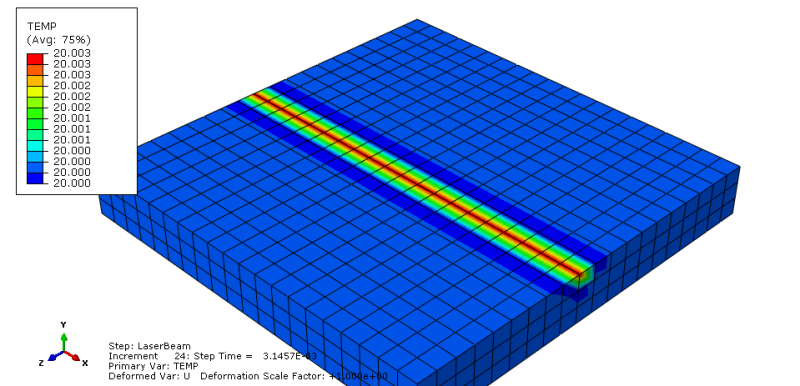The temperature values are very small. Additionaly, above results refer to very small step time value. At the end of the simulation, there is no heat flux effect: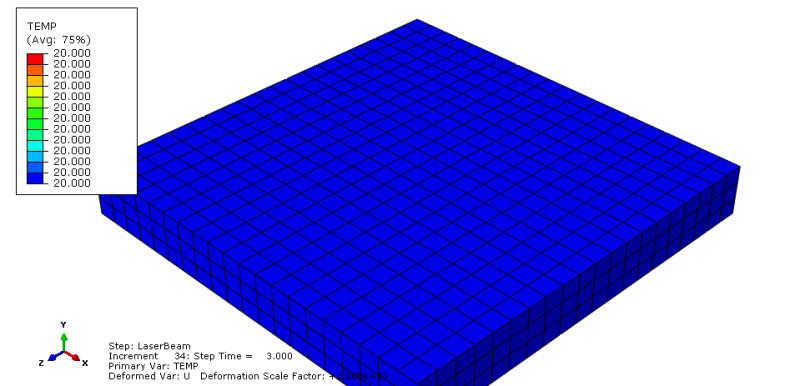Then I modified the code:

#### CODE --> Fortran

SUBROUTINE DFLUX(FLUX,SOL,KSTEP,KINC,TIME,NOEL,NPT,COORDS,
1 JLTYP,TEMP,PRESS,SNAME)
C
INCLUDE 'ABA_PARAM.INC'
C
DIMENSION FLUX(2), TIME(2), COORDS(3)
CHARACTER*80 SNAME

QL=120000.d0                        !LASER POWER [mW]
DEPTH=0.5d0                         !PENETRATION DEPTH [mm]
Q=(QL/(R0**2*3.1415926d0*DEPTH))    !BODY FLUX [mW/mm^3] ?
!     V=5.d0                              !LASER BEAM VELOCITY [mm/s]

X0=-13.75d0                         !STARTING COORDINATE OF THE LASER BEAM
Y0=-7.5d0                           !STARTING COORDINATE OF THE LASER BEAM
Z0=11.d0                            !STARTING COORDINATE OF THE LASER BEAM

X=COORDS(1)
Y=COORDS(2)
Z=COORDS(3)

IF(X.EQ.X0 .AND. Z.EQ.Z0) THEN
FLUX(1)=Q
ELSE
FLUX(1)=0
END IF

RETURN
END 

And I got: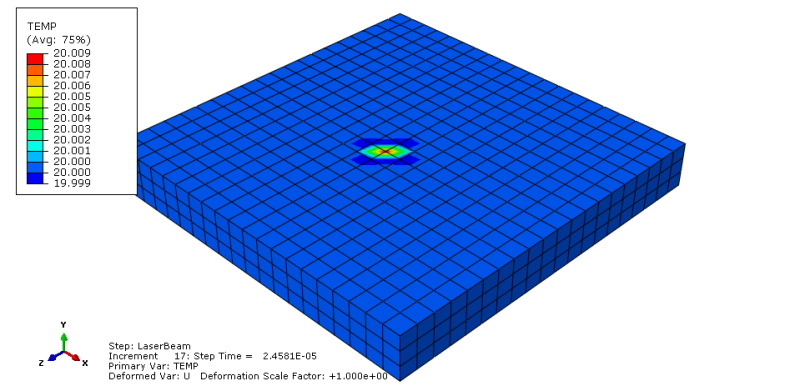It looks good, but the temperature values are very low. Again these results refer to small step time. When it grows, the heat flux effect dissapears.

What about Y coordinates? Should I define them? In a load module, as a picked region, I have chosen the top surface. There is one Y coordinate for the top surface.

How can I change the code to obtain correct results?
I attach my CAE file. Maybe it will be helpful.
CAE: http://files.engineering.com/getfile.aspx?folder=3...

### RE: Moving Heat Flux (laser) - From the simplest example - DFLUX subroutine

(OP)
I refresh the topic.

### RE: Moving Heat Flux (laser) - From the simplest example - DFLUX subroutine

Are you aware that you just get energy entered, when an integration point of an element surface is exactly at your X0 and Z0 coordinates?

### RE: Moving Heat Flux (laser) - From the simplest example - DFLUX subroutine

(OP)
Yes, but this energy enters right at the beginning of the step. Next, it disappears. Why it disappears?

### RE: Moving Heat Flux (laser) - From the simplest example - DFLUX subroutine

Maybe the integration point moves a little bit because of thermal expansion. But only if you do a fully-coupled analysis.

### RE: Moving Heat Flux (laser) - From the simplest example - DFLUX subroutine

(OP)
You were right Mustaine3. I removed the thermal expansion coefficient and it works very good now.
So what is the best way to control the area, on which the laser beam works?

I thought of using the followng code:

#### CODE --> Fortran

SUBROUTINE DFLUX(FLUX,SOL,KSTEP,KINC,TIME,NOEL,NPT,COORDS,
1 JLTYP,TEMP,PRESS,SNAME)
C
INCLUDE 'ABA_PARAM.INC'
C
DIMENSION FLUX(2), TIME(2), COORDS(3)
CHARACTER*80 SNAME

QL=120000.d0                        !LASER POWER [mW]
DEPTH=0.5d0                         !PENETRATION DEPTH [mm]
Q=(QL/(R0**2*3.1415926d0*DEPTH))    !BODY FLUX [mW/mm^3] ?
!     V=5.d0                              !LASER BEAM VELOCITY [mm/s]

X0=-13.75d0                         !STARTING COORDINATE OF THE LASER BEAM
Y0=-7.5d0                           !STARTING COORDINATE OF THE LASER BEAM
Z0=11.d0                            !STARTING COORDINATE OF THE LASER BEAM

X1=-12.75d0
Z1=-6.5d0

X=COORDS(1)
Y=COORDS(2)
Z=COORDS(3)

IF(X.GT.X0 .AND. X.LT.X1 .AND. Z.GT.Z0 .AND. Z.LT.Z1) THEN
FLUX(1)=Q
ELSE
FLUX(1)=0
END IF

RETURN
END 

But this code doesn't work. How can I make it work?
And why the values of heat flux density are so huge?: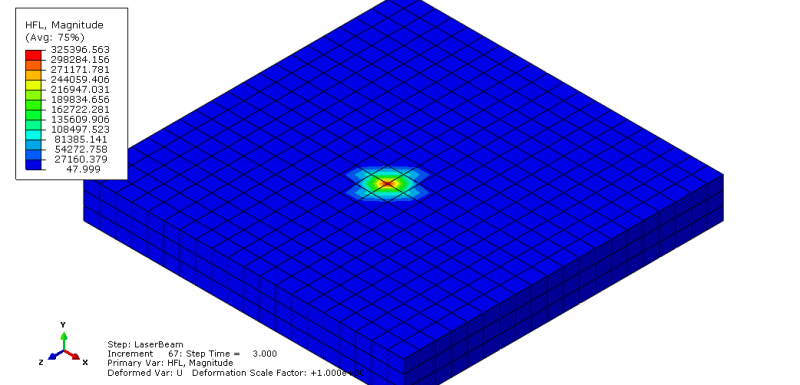### RE: Moving Heat Flux (laser) - From the simplest example - DFLUX subroutine

(OP)
I refresh the topic again.
How can I expand the heat flux affected area?

### RE: Moving Heat Flux (laser) - From the simplest example - DFLUX subroutine

Check your IF formula again. I think it will never be fulfilled with your data.

### RE: Moving Heat Flux (laser) - From the simplest example - DFLUX subroutine

(OP)
You are right, but I know, that IF formula is a problem.
I do not have an idea how to modify it to obtain desired expanded heat flux affected area.

### RE: Moving Heat Flux (laser) - From the simplest example - DFLUX subroutine

(OP)
I did few mistakes in that subroutine.

X0=-13.75d0
Y0=-7.5d0
Z0=11.d0
X1=-12.75d0
Z1=-6.5d0

#### CODE --> Fortran

IF(X.GT.X0 .AND. X.LT.X1 .AND. Z.GT.Z0 .AND. Z.LT.Z1) THEN

In that formula I have an operator .GT. an .LT.
So, the X0 and X1 values are not taken into account. There are no nodes (first coordinate of the nodes) between X0 and X1, because the distance between two nodes equals 1.

The next mistake concerns the Z coordinate:
I have: Z.GT.Z0
It should be: Z.LTZ0

I have: Z.LT.Z1
It should be: Z.GT.Z1

So, the final subroutine is:

#### CODE --> Fortran

SUBROUTINE DFLUX(FLUX,SOL,KSTEP,KINC,TIME,NOEL,NPT,COORDS,
1 JLTYP,TEMP,PRESS,SNAME)
C
INCLUDE 'ABA_PARAM.INC'
C
DIMENSION FLUX(2), TIME(2), COORDS(3)
CHARACTER*80 SNAME

QL=120000.d0                        !LASER POWER [mW]
DEPTH=0.5d0                         !PENETRATION DEPTH [mm]
Q=(QL/(R0**2*3.1415926d0*DEPTH))    !BODY FLUX [mW/mm^3] ?
!     V=5.d0                              !LASER BEAM VELOCITY [mm/s]

X0=-13.75d0                         !STARTING COORDINATE OF THE LASER BEAM
Y0=-7.5d0                           !STARTING COORDINATE OF THE LASER BEAM
Z0=11.d0                            !STARTING COORDINATE OF THE LASER BEAM

X1=-12.75d0
Z1=10.d0

X=COORDS(1)
Y=COORDS(2)
Z=COORDS(3)

IF(X.GE.X0 .AND. X.LE.X1 .AND. Z.LE.Z0 .AND. Z.GE.Z1) THEN
FLUX(1)=Q
ELSE
FLUX(1)=0
END IF

RETURN
END 

And the result is: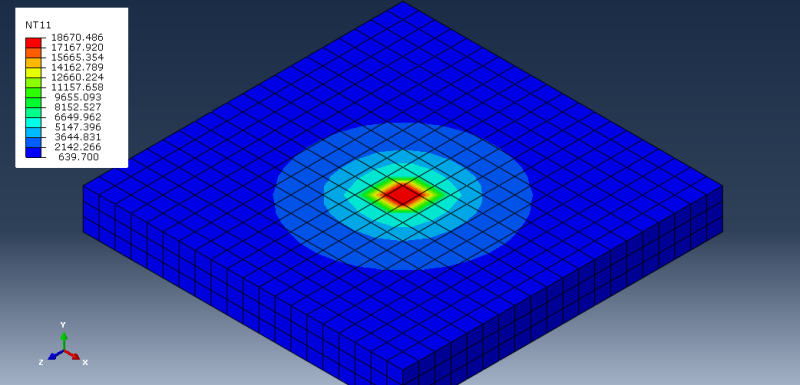The next step will be creating the volumetric heat flux. The aim is to create the Gaussian Distribution.
Now I will try to do this alone and I will ask for help if I will have some problems.

### RE: Moving Heat Flux (laser) - From the simplest example - DFLUX subroutine

Thank you very much for your post. It is very useful. I met one problem when I use DFLUX. I tried to simulate one heat source moves as a curve. The heat source moving track is parabola, here is the function: y=x(x-1) with the constant speed of 0.002m/s. Do you have any ideas on this? Thanks.

### RE: Moving Heat Flux (laser) - From the simplest example - DFLUX subroutine

Hey BruceZhang2010,

Were you able to include moving heat into your fortran code? I tried to do something similar, but it doesn't really work. Could you maybe send an idea?
Thanks

#### Red Flag This Post

Please let us know here why this post is inappropriate. Reasons such as off-topic, duplicates, flames, illegal, vulgar, or students posting their homework.

#### Red Flag Submitted

Thank you for helping keep Eng-Tips Forums free from inappropriate posts.
The Eng-Tips staff will check this out and take appropriate action.

#### Resources

Research Report - Do Engineers Need On-Demand Manufacturing for Additive and Subtractive Processes?
Manufacturing professionals are beginning to explore digital manufacturing technologies in their efforts to reduce cost and improve quality and efficiency of parts and processes. Additive manufacturing is one digital technology which is changing the way we think about the design and life-cycle of manufactured parts. Download Now
White Paper - Considerations for choosing a 3D printing technology
The adoption of 3D printing into major companiesâ€™ product development life cycles is a testament to the technologyâ€™s incredible benefits to consumers, designers, engineers and manufacturers. While traditional production methods have limitations in manufacturability, 3D printing provides unparalleled design freedom due to the additive method of building parts layer by layer. Download Now
eBook - Manufacturing solutions across the product development life cycle
In this ebook, we discuss five stages of the product development life cycle and key services that help engineers and designers move forward effectively. Stratasys Direct Manufacturingâ€™s advanced manufacturing solutions can help your team accomplish its goals quickly and with ease. Download Now
White Paper - Common pitfalls to avoid when prototyping with 3D printing
Keeping your application and its intended use in mind is key when prototyping. For example, early in the design process, focusing too greatly on creating components or products specifically for the additive manufacturing (3D printing) process used for prototyping may cause challenges down the road. Download Now

Close Box

# Join Eng-Tips® Today!

Join your peers on the Internet's largest technical engineering professional community.
It's easy to join and it's free.

Here's Why Members Love Eng-Tips Forums:

•Talk To Other Members
• Notification Of Responses To Questions
• Favorite Forums One Click Access
• Keyword Search Of All Posts, And More...

Register now while it's still free!